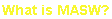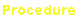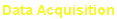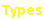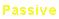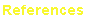e-mail: contact@masw.com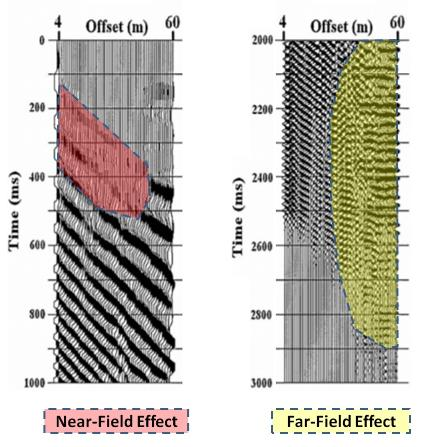(Above) Figure 1.  A schematic illustrating the near- and far-field effects that can prevail during
an MASW survey.  The offset range free of these two effects is called an "optimum" offset.
(Below) Figure 2.  A field record obtained by using a 12-lb sledge hammer source.  Original
record is stretched (convolved) with a sweep function varying from 5 Hz to 50 Hz, and the low
(5-20 Hz) and high (35-50 Hz) frequency parts are displayed here to show the portions
contaminated by the near- and far-field effects, respectively.
distance from the source (i.e., offset) where surface waves are
best developed with minimal inclusion of adverse influences.
There are two factors that may impede the best development;
the near- and far-field effects.

Near-Field Effects

Surface waves are not developed at the vicinity of source point
because they are formed through interference of (P and SV)
body waves generated from reflections and refractions,
occurrence of which require a certain minimum distance from
the source.  Therefore, the first receiver closest to the source
should be placed beyond this point, and this is called the near-
field effects.  If a receiver is placed within this distance, it will
record either body or ambient noise wavefields.

This minimum distance changes with wavelength; a longer
wavelength needs a longer distance to be fully developed.  A
rule of thumb is 25-50% of the wavelength.  However, an MASW
survey deals with a wide range of wavelengths (e.g., 1-50 m),
and this range is determined by the receiver spacing (dx) (e.g.,
dx=1 m) and length of the receiver array (L) (e.g., L=50 m).  In
practice, therefore, one distance compromising the entire range
of wavelengths is used to set the minimum distance between
the source and the closest receiver, and this is called the
source offset (X1) (Figure 1).  It is usually between 25% and
50% of the receiver array length (L):

0.25L <= X1 <= 0.5L                                  (1)

Far-Field Effects

Although surface waves are much stronger than body waves at
the time of generation near the source point, they attenuate
more rapidly as illustrated in Figure 1.  In consequence, after
travelling a certain distance, their energy level may drop below
that of the body waves or ambient noise.  It is, therefore,
important to place receivers closer than this maximum distance
(Xmax) to avoid the contamination by these noise wavefields.
This is called the far-field effects.  Xmax depends on (1) source
power, (2) ambient noise level, and (3) attenuation property of
subsurface.  The first, (1), acts positively, whereas the other
two, (2) and (3), act negatively in increasing Xmax.  As a rule of
thumb for MASW surveys using a heavy sledge-hammer source
(e.g., 16-lb), Xmax is about 50 m in typical urban areas with
heavy traffic, while it can be extended to 100 m at relatively
quiet areas.  A more powerful source (e.g., weight drop) can
extend Xmax by, for example, 30%.

Both near- and far-field effects are illustrated in a field record
(Figure 2) obtained by using a 12-lb sledge hammer source.
The original field record has been stretched (i.e., convolved)
with monotonically increasing frequency function (i.e., sweep) in
5-50 Hz.  At the lowest frequency of 5 Hz that corresponds to
the longest wavelength, surface waves are not effectively
developed at near offsets (e.g., < 40 m) due to the near-field
effects (left panel in Figure 2), while body waves and ambient
noise dominate at the far offsets (e.g., > 30 m) due to the far-
field effects (right panel in Figure 2).